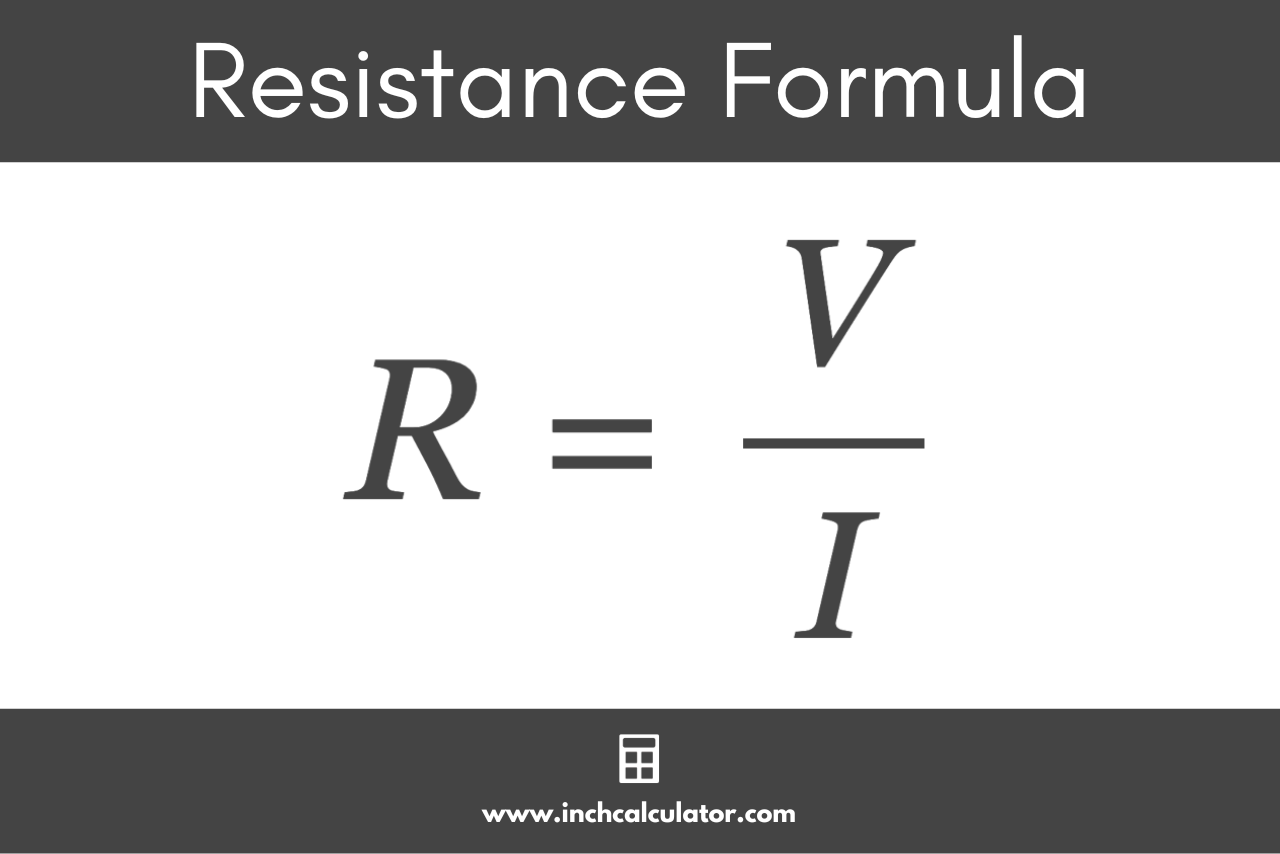# Resistance Calculator

Use the resistance calculator below to calculate the resistance in ohms given the voltage, current, or power.

## Calculation Formulas:

Learn how we calculated this below

## How to Calculate Electrical Resistance

In an electrical circuit, resistance is the force that opposes the flow of current through the circuit or conductor. Resistance is measured in ohms (Ω).

You can calculate the resistance in a circuit using Ohm’s Law, which states that the current through a circuit element is directly proportional to the potential difference applied to it and inversely proportional to the resistance.

### Resistance Formula

The Ohm’s Law formula is I = V/R, where I is the current through the circuit (in amps), V is the potential difference across the circuit (in volts), and R is the resistance of the circuit (in ohms).

By rearranging this formula, you can calculate the resistance by dividing the voltage by the current. The formula to calculate resistance is:

R = V / I

Thus, the resistance of the circuit R is equal to the voltage across the circuit V divided by the current flowing through the circuit I.## How to Calculate the Resistance Using Watts

You can also calculate the resistance in a circuit using Watt’s Law power formula. If you know the power in watts drawn through a circuit and the current, the formula to calculate the resistance is:

R = P /

The resistance of the circuit R is equal to the power drawn through the circuit P divided by the current flowing through the circuit I squared.

Alternatively, if you know the power drawn through the circuit and the voltage, then you can calculate the resistance using the formula:

R = / P

The resistance of the circuit R is equal to the potential difference across the circuit V squared divided by the power drawn through the circuit P.

## References

1. Brandon Mitchell, Robert Ekey, Roy McCullough, and William Reitz, A Fan-tastic Quantitative Exploration of Ohm’s Law, https://aapt.scitation.org/doi/full/10.1119/1.5021431
2. National Institute of Standards and Technology, Ampere: The Present, https://www.nist.gov/si-redefinition/ampere-present